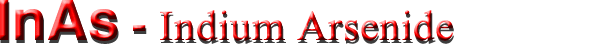## Optical properties

 Infrared refractive index ≈3.51 (300 K) Radiative recombination coefficient 1.1·10-10 cm3/s Long-wave TO phonon energy hνTO ≈27 meV (300 K) Long-wave LO phonon energy hνLO ≈29 meV (300 K)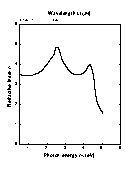Refractive index n versus photon energy. Solid curve is theoretical calculation. Points represent experimental data, 300 K. (Adachi).
For 3.75 µm < λ < 33 µm
n = [11.1 + 0.71/(1-6.5·λ-2) + 2.75/(1-2085·λ-2) - 6·10-4·λ2)]1/2,
where λ is the wavelength in µn (300 K)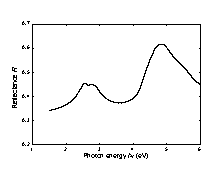Normal incidence reflectivity versus photon energy, 300 K (Aspnes and Studna ).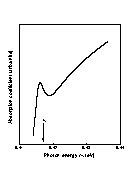Absorption coefficient near the intrinsic absorption edge for n-InAs. T=4.2 K (Varfolomeev et al. ).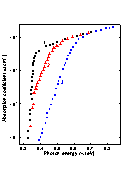Absorption coefficient versus photon energy for different donor concentration, 300 K n (cm-3): 1. 3.6·1016, 2. 6·1017, 3. 3.8·1018. (Dixon and Ellis ).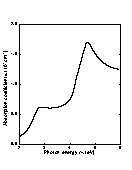Absorption coefficient versus photon energy, T = 300 K (Aspnes and Studna).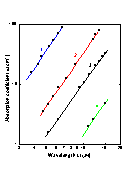Free carrier absorption versus wavelength at different electron concentrations. T=300 K. no (cm-3): 1. 3.9·1018; 2. 7.8·1017; 3. 2.5·1017; 4. 2.8·1016; (Dixon ).